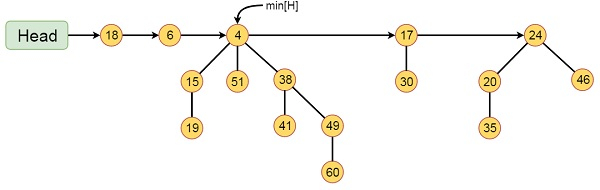# Fibonacci Heaps in Data Structure

Like Binomial heaps, Fibonacci heaps are collection of trees. They are loosely based on binomial heaps. Unlike trees with in binomial heaps are ordered trees within Fibonacci heaps are rooted but unordered.

Each node x in Fibonacci heaps contains a pointer p[x] to its parent, and a pointer child[x] to any one of its children. The children of x are linked together in a circular doubly linked list known as child list of x. Each child y in a child list has pointers left[y] and right[y] to point left and right siblings of y respectively. If node y is only child then left[y] = right[y] = y. The order in which sibling appears in a child list is arbitrary.

## Example of Fibonacci HeapThis Fibonacci Heap H consists of five Fibonacci Heaps and 16 nodes. The line with arrow head indicates the root list. Minimum node in the list is denoted by min[H] which is holding 4.

The asymptotically fast algorithms for problems such as computing minimum spanning trees, finding single source of shortest paths etc. makes essential use of Fibonacci heaps.